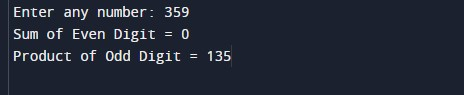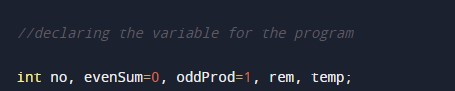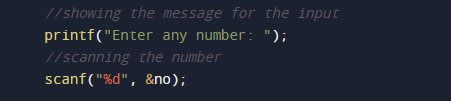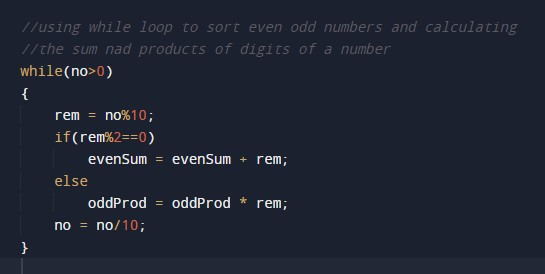C Program to Print Sum of Even & Product of Odd Digit

In this tutorial you will learn about the C Program to Print Sum of Even & Product of Odd Digit and its application with practical example.

C Program to Print Sum of Even & Product of Odd Digit

In this tutorial, we will learn to create a C program that will Print Sum of Even & Product of Odd Digit using C programming.

Prerequisites

Before starting with this tutorial we assume that you are best aware of the following C programming topics:

• Operators in C Programming.
• Basic Input and Output function in C Programming.
• Basic C programming.
• While loop in C programming.
• Arithmetic operations in C Programming.

Program to Print Sum of Even & Product of Odd Digit

In c programming, it is possible to take numerical input from the user and Print Sum of Even & Product of Odd Digit with the help of a very small amount of code. The C language has many types of header libraries which has supported function in them with the help of these files the programming is easy.

With the help of this program, we can Print Sum of Even & Product of Odd Digit.

Output:-In the above program, we have first initialized the required variable.• evenSum = it will hold the integer value for the sum of numbers.
• oddProd = it will hold the integer value for the product of the numbers.
• temp = it will hold the temporary integer value.
• rem = it will hold the integer value of the remainder.
• no = it will hold the integer value of the input.

Input number from the user.Program Logic Code.Printing output for sum and the product.In this tutorial we have learn about the C Program to Print Sum of Even & Product of Odd Digit and its application with practical example. I hope you will like this tutorial.